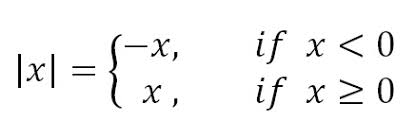Absolute Difference Calculator

The absolute value of a number represents the distance between 0 and the number on the number line.

Absolute Value or Absolute Difference Definition

The difference between two numbers a and b is the absolute value of their difference |a − b|. It makes no difference which number we designate by “a” and which number we designate by “b”.

Calculate the Absolute Difference i.e. absolute value of the two numbers here.

Absolute Value

The absolute value of X is written as |X|

Because we are taking the absolute value of the difference between the numbers, we will always end up with a positive number and order will not matter.

Absolute Difference Formula